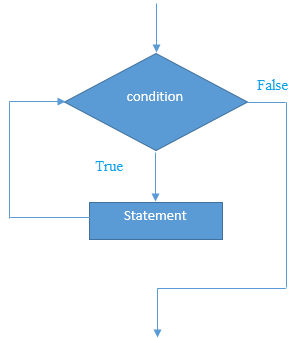# C++ while循环

```while(condition){
//code to be executed
}
```

while循环的执行流程图-C++ while循环示例

```#include <iostream>
using namespace std;
int main() {
int i=1;
while(i<=10)
{
cout<<i <<"\n";
i++;
}
return 0;
}
```

```1
2
3
4
5
6
7
8
9
10```

C++嵌套While循环示例

```#include <iostream>
using namespace std;
int main () {
int i=1;
while(i<=3)
{
int j = 1;
while (j <= 3)
{
cout<<i<<" "<<j<<"\n";
j++;
}
i++;
}
return 0;
}
```

```1 1
1 2
1 3
2 1
2 2
2 3
3 1
3 2
3 3
```

C++无限循环示例：

```#include <iostream>
using namespace std;
int main () {
while(true)
{
cout<<"Infinitive While Loop";
}
return 0;
}
```

```Infinitive While Loop
Infinitive While Loop
Infinitive While Loop
Infinitive While Loop
Infinitive While Loop
ctrl+c```